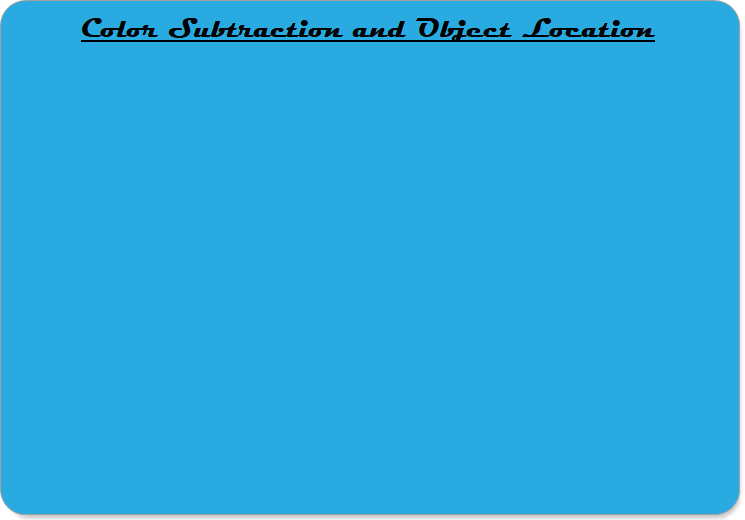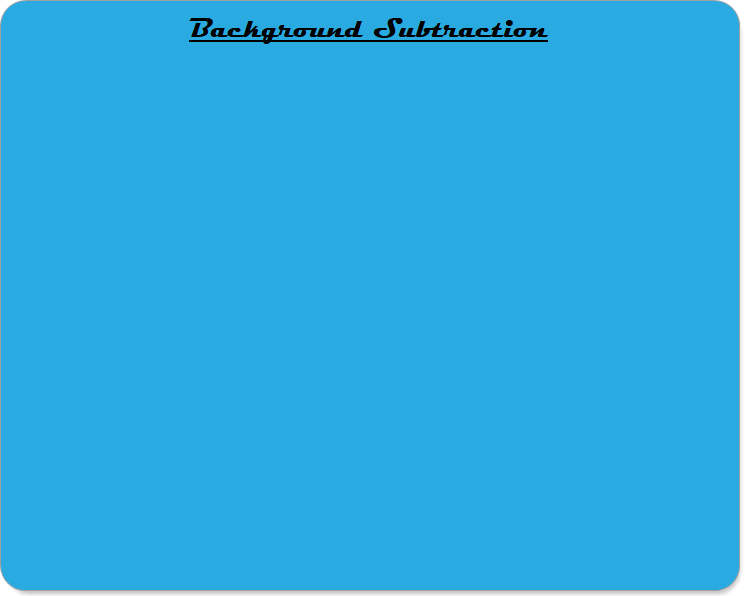Robotics 1
Image SubtractionThis video shows how color images can be subtracted to isolate a single object of a particular color as a bright object on a dark background.  Then, we learn the 'center of mass' approach to finding the location of the object in the image, in units of 'pixels'.This video shows another application of image subtraction: background subtraction.  Whereas color subtraction is useful for isolating an object of a particular color, background subtraction is useful for isolating an object that either enters or leaves the camera field of view.  In this video, we also learn the difference between a 'grayscale' image and a 'black and white' image.  We use the method of 'thresholding' to reduce image noise by converting a grayscale image to a black and white image.
To complete this lab activity, make a video that includes the following in one video:

(1) You saying your name
(2) You moving a red object in the view of the camera while showing the column location output from Python
(3) You demonstrating the output of your background subtraction code, with the Python output showing BOTH the column location AND the row location of the object.  Note that this will require you to add some code not shown in the video to find the row location.  Hint: after you sum the rows, you will have a column vector which you need to transpose into a row vector (see https://www.tutorialspoint.com/numpy/numpy_transpose.htm)

Graduate students also have to show the following:
(4) The output of the Python code tracking the motion of your hand moving across the screenIn this video, we are learning about one approach to motion detection known as 'motion by difference'.  In this method, we capture two images spaced in time by an amount 'delta t' and take the difference of the two images to find only what has changed between the two images.